Merge Multiple Cells into One Cell with VBA

This post will guide you how to combine multiple cells into one cell with VBA code in Excel. How do I concatenate a range of cells with VBA macro in Excel 2013/2016. How to merge the contents of two or multiple cells into one cell with macro in Excel.

Merge multiple Cells into One Cell with VBA

If you want to merge multiple cells into one cell without losing data in excel, you can try to use an Excel VBA macro to quickly achieve the result. Just do the following steps:

#1 open your excel workbook and then click on “Visual Basic” command under DEVELOPER Tab, or just press “ALT+F11” shortcut.#2 then the “Visual Basic Editor” window will appear.

#3 click “Insert” ->”Module” to create a new module#4 paste the below VBA code into the code window. Then clicking “Save” button.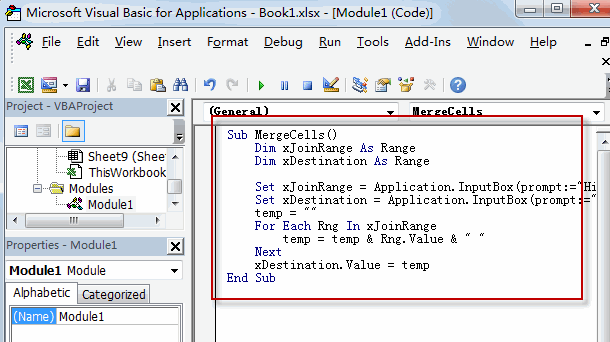Sub MergeCells()
Dim xJoinRange As Range
Dim xDestination As Range

Set xJoinRange = Application.InputBox(prompt:="Highlight source cells to merge", Type:=8)
Set xDestination = Application.InputBox(prompt:="Highlight destination cell", Type:=8)
temp = ""
For Each Rng In xJoinRange
temp = temp & Rng.Value & " "
Next
xDestination.Value = temp
End Sub

#5 back to the current worksheet, then run the above excel macro. Click Run button.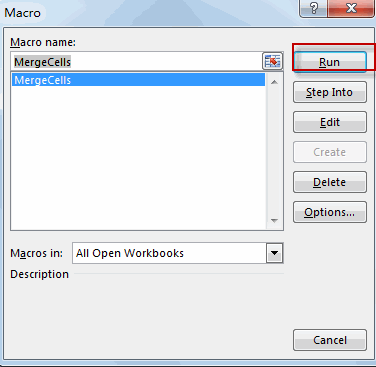#6 select one range of cells to merge cells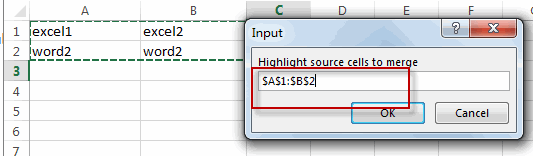#7 select one destination cell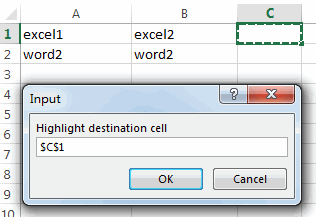#8 let’s see the result.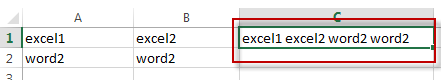You can also write an user defined function with VBA to merge multiple cells in Excel. just do use the following vba code:

Function MergeCells(sourceRange As Excel.Range) As String

Dim finalValue As String

Dim cell As Excel.Range

For Each cell In sourceRange.Cells

finalValue = finalValue + CStr(cell.Value)

Next cell

MergeCells = finalValue

End Function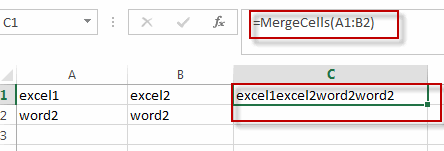Related Posts

VBA Macro For VLOOKUP From Another Sheet

In the previous post, you should know that how to fix or remove the #N/A error when using VLOOKUP formula to lookup value from another sheet. And this post will show you how to use VBA code to vlookup data ...

How To Insert Comments in Protected Worksheet in Excel

This post will show you how to allow comments in a protected worksheet in Excel. You can easily to insert comments into cells in a normal worksheet in Excel, but if want to insert a comment in a worksheet that ...

How To Convert Text to Upper Cases(Using VBA) in Excel

This post will show you how to switch from lower case to upper case in Excel. and I am going to show you two different ways of converting text to upper cases using formula or VBA macro in Excel 2013,Excel ...

How To Hide Every Other Row in Excel (Using VBA)

This post will show you how to hide alternate rows or columns in Excel or how to hide every third, fourth, fifth row or column in Excel. If you want to hide every other row in your current worksheet, how ...

How to Disable the Save As Prompt in Excel

This post will show you how to use a VBA Macro to save an Excel file and overwrite any existing file without a prompt so that you are going to get the little window that says file already exists do ...

How to Count Cells that Contain even or odd numbers in Excel

This post will guide you how to count the number of cells that contain odd or even numbers within a range of cells using a formula in Excel 2013/2016.How do I count cells that contain odd numbers through the use ...

How to Count Cells that Contain negative Numbers in Excel

This post will guide you how to count the number of cells that contain negative numbers within a range of cells using a formula in Excel 2013/2016.You can count the number of negative numbers in your data using easy functions ...

How to Count Cells Are Not Blank or Empty in Excel

This post will guide you how to count cells that are not blank or empty in a given range cells using a formula in Excel 2013/2016.How do I count the number of cells that are not blank in a particular ...

How to Count Cells Less Than a Specific Value in Excel

This post will guide you how to count the number of cells less than a particular numeric value in a given range cells using a formula in Excel 2013/2016. How do I count cells that are less than a specific ...

How to Count Cells Greater Than a Specific Value in Excel

This post will guide you how to count the number of cells greater than a particular numeric value in a given range cells using a formula in Excel 2013/2016. How do I count cells that are greater than a specific ...

Sidebar